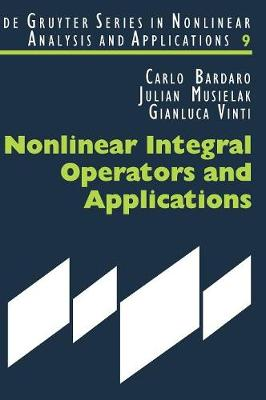•Nonlinear Integral Operators and Applications - De Gruyter Series in Nonlinear Analysis & Applications (Hardback)

(author), (author), (author)
£136.50
Hardback 213 Pages / Published: 19/06/2003
• We can order this

Usually dispatched within 3 weeks

In 1903 Fredholm published his famous paper on integral equations. Since then linear integral operators have become an important tool in many areas, including the theory of Fourier series and Fourier integrals, approximation theory and summability theory, and the theory of integral and differential equations. As regards the latter, applications were soon extended beyond linear operators. In approximation theory, however, applications were limited to linear operators mainly by the fact that the notion of singularity of an integral operator was closely connected with its linearity. This book represents the first attempt at a comprehensive treatment of approximation theory by means of nonlinear integral operators in function spaces. In particular, the fundamental notions of approximate identity for kernels of nonlinear operators and a general concept of modulus of continuity are developed in order to obtain consistent approximation results. Applications to nonlinear summability, nonlinear integral equations and nonlinear sampling theory are given. In particular, the study of nonlinear sampling operators is important since the results permit the reconstruction of several classes of signals. In a wider context, the material of this book represents a starting point for new areas of research in nonlinear analysis. For this reason the text is written in a style accessible not only to researchers but to advanced students as well.

Publisher: De Gruyter
ISBN: 9783110175516
Number of pages: 213
Weight: 494 g
Dimensions: 240 x 170 x 17 mm